How to draw lines in TradingView pinescript?

There are 3 ways that I know of on how to draw lines in TradingView  pinescript. And, these are:
• hline()
• plot()
• line.new()

hline()

The hline() or Levels are used to plot horizontal lines across the chart. It is commonly used in RSI to indicate the oversold and overbought levels.

Let's say we have an oscillator that is very similar to RSI and we want to plot the oversold and overbought levels,  we can do that easily by calling the hline function:
hline(price, title, color, linestyle, linewidth, editable) → hline
And here's the example of trying to use the function:

And here's the result when plotted to chart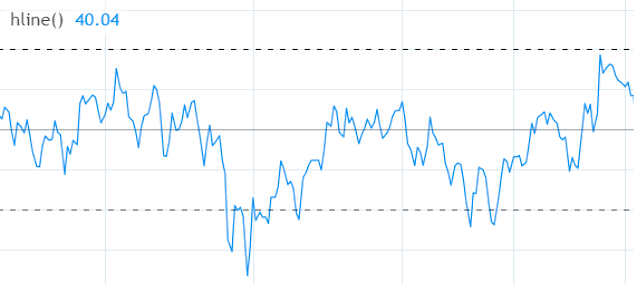RSI + Levels (30, 50, 70)

plot()

The plot() function is often used to draw almost all lines in TradingView pinescript. We can plot many things from a wave-like line to a straight line.

For example we want to plot a Simple Moving Average on our chart: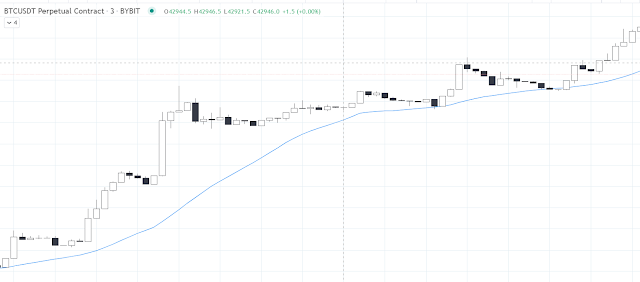Plotting a Simple Moving Average using plot()

What if let's say we want the ability to draw major support and resistances on our chart? Then we can also use the plot() for that. Here's an example:

Then let's change the settings of our Support and Resistance indicator to this: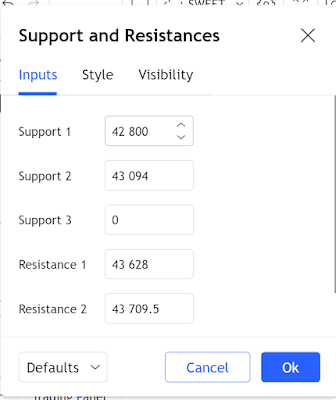Support and Resistance Indicator Settings

Then let's check the chart to see what happened...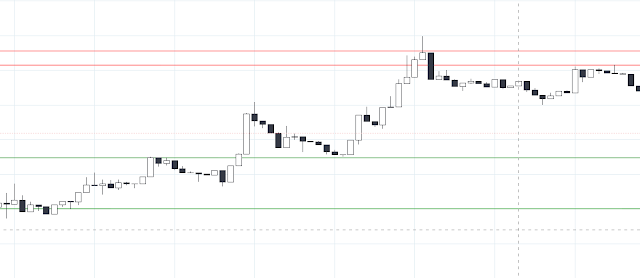Support and Resistance Indicator using plot()

Now, we can draw major support and resistances with this indicator so that we always know the important levels of the market. Also, this is the same result as hline, so you can use them too if you want. In fact, when drawing straight lines i prefer  to use the hline.

line.new()

The line.new() is mostly used to draw line segments and zigzags over the chart. You can even draw a ray line that extends to the right or left, depending on the parameter that is set.

One drawback for using this function is that TradingView limits the total number of drawings of this because it might consume server resources. And because of that TradingView delete's the old drawings of this lines and replaces it with the most recent ones.

For this one, let's say we want to draw a ray line starting from the highest highs on the most recent 30 days. And the line should extend to the right, acting as resistance.

We can do that by:

Now, if you look at the picture below, we have a nice-looking ray that acts as immediate resistance.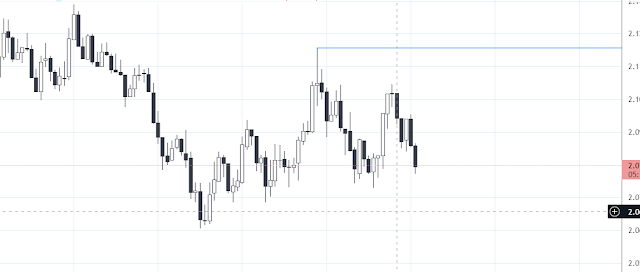Nice-Looking Resistance using line.new()

Conclusion

As you can see, there are many different ways to draw lines in TradingView and each of them has a different use.

My advise is to use them when:

plot() - as much as possible use this one as this one have no limits compared to line.new(). Use this one for non-straight lines.

hline() - drawing levels for oscillators like RSI.

line.new() - drawing line.segments or lines that you need to modify because this one is deletable and updateable.

With these 3 we can now draw any kind of lines that we may need to complete our trading strategy. We can draw profit targets, stop loss targets, zigzags, support and resistances, and many more. You can even draw important support and resistance so that you have one glance look every time you are checking the chart.

A Little Favor From You 😅

Since I give everything for free in this blog, please have time to support the blog by following or subscribing to some of my social media accounts:

👉 Blog